# Google Sheets IF function - usage and formula examples

The IF function in Google Sheets is one of the easiest functions to learn, and while this holds true, it is also a very helpful one.

In this tutorial, we will take a closer look at how Google Spreadsheet IF function works and what advantages we can get from using it.

## What is IF function in Google Sheets?

Whenever we use IF function, we create a decision tree in which certain action follows under one condition, and if that condition is not met - another action follows.

For this purpose, the condition of the function must be in a format of the alternative question with only two possible answers: "yes" and "no".

This is how a decision tree may look like:So, IF function allows us to ask a question and indicate two alternative actions depending on the received answer. This question and the alternative actions are known as three arguments of the function.

## IF function syntax in Google Sheets

The syntax for the IF function and its arguments are as follows:

=IF(logical_expression, value_if_true, value_if_false)
• logical_expression - (required) a value or logical expression that is tested to see if it is TRUE or FALSE.
• value_if_true - (required) the operation that is carried out if the test is TRUE.
• value_if_false - (optional) the operation that is carried out if the test is FALSE.

Let's explore the arguments of our IF function in more detail.

The first argument represents a logical question. Google Sheets answers this question with either "yes" or "no", i.e. "true" or "false".

How to formulate the question properly, you may wonder? To do that, you can write a logical expression using such helpful symbols as "=", ">", "<", ">=", "<=", "<>". Now we'll try and ask such a question together.

## Usage of the IF function

Let's assume that you are working in the company which sells chocolate in several consumer regions and works with many clients.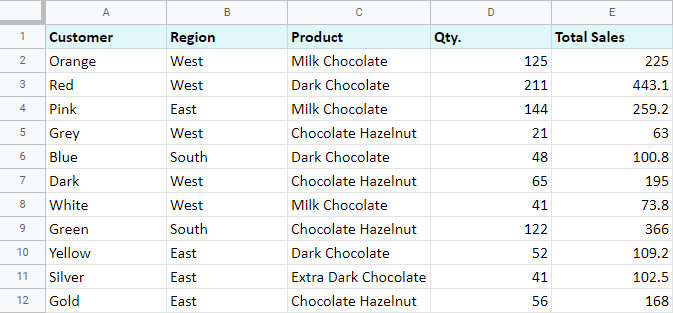We need to separate sales which were made in our local regions from those which were made abroad. To accomplish that, we should add another descriptive field for each sale - a country where the sales took place. Since we have lots of data, we want this description field to be created automatically for each entry.

And this is when IF function comes to play. Let's add the "Country" column to the data table. "West" region represents local sales (Our Country), while the rest are the sales from abroad (Rest of the World).

## How to write out the function properly?

Place the cursor in F2 to make the cell active and type in the equality sign (=). Google Sheets will immediately understand that we are going to enter a formula. That's why right after we type the letter "i" it will prompt us to choose a function which begins with that same letter. And we choose "IF".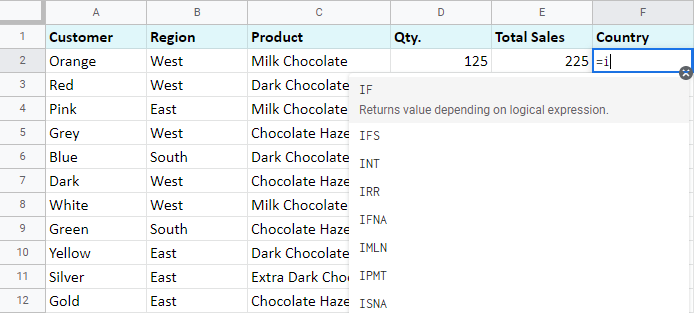After that, all our actions will be accompanied by prompts as well.

For the first argument of the IF function, enter: B2="West". As with the other Google Sheets functions, you don't need to enter the address of the cell manually - mouse click is enough. Then enter comma (,) and specify the second argument.

The second argument is a value that F2 will return if the condition is met. In this case, it will be a text "Our Country".

And again, after the comma write in the value of the 3rdargument. F2 will return this value if the condition is not met: "Rest of the World". Do not forget to finish your formula entry by closing parenthesis ")" and pressing "Enter".

Our function should look like this:

`=IF(B2="West","Our Country","Rest of the World")`

And F2 returns the text "Our Country".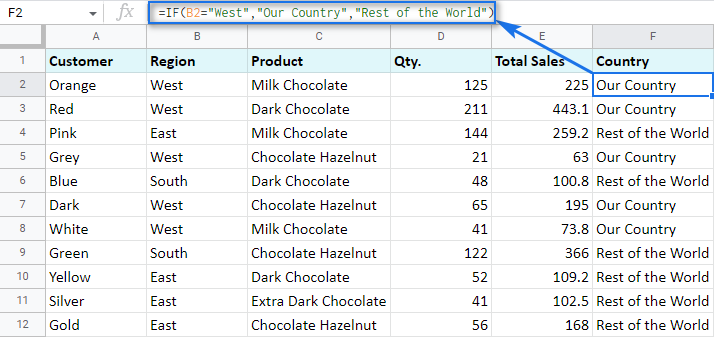Now, all we have to do is to copy this function down the column F.

Let's examine the other ways of working with IF function.

## IF function and text values

The usage of the IF function with a text has already been illustrated in the example above.

Note. Please pay attention that if the text is being used as the argument, then it must be enclosed in double quotes.

## IF function and numerical values

We can use numbers for the arguments just as we did it with the text.

However, what is very important here is that the IF function makes it possible to not only fill cells with certain numbers based on the conditions met but also calculate.

For example, let's say we give our clients discounts based on the total value of the purchase. If the total is more than 200, then the client receives a 10% discount.

For that, we will use column G and name it "Discount". Then enter IF function in G2, and the second argument will be represented by the formula which calculates the discount:

`=IF(E2>200,E2*0.1,0)`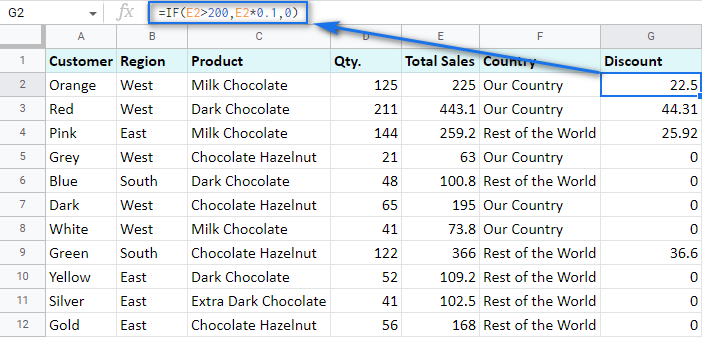## IF in combination with other functions

As we have already learned, the text, numbers, and formulas can act as the arguments of the IF function. However, other functions can play that role as well. Let's see how it works.

Remember the way we figured out the country where we sold chocolate?

Now we can use OR function as a first argument, which will return "YES" if at least one of the listed conditions is true. We simply change the IF function in the cell F2. For the first argument, we will list all the regions which belong to the sales abroad, and the other sales will automatically become local.

`=IF(OR(B2="East",B2="South"),"Rest of the World","Our Country")`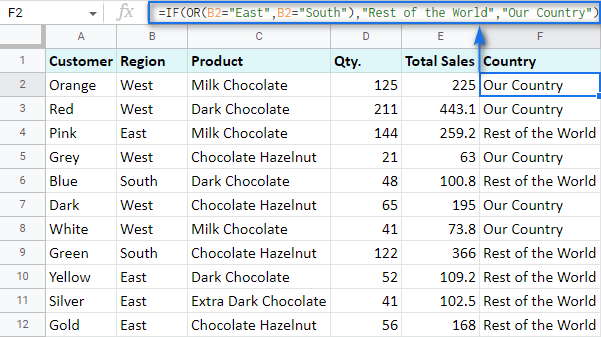We can also use the IF function itself as an argument for the bigger IF function.

Let's assume that we have set stricter discount conditions for our clients. If the total purchase is more than 200 units then they get a 10% discount; if the total purchase is between 100 and 199 then the discount is 5%. If the total purchase is lower than 100, there is no discount whatsoever.

The following formula shows how the function will look in the cell G2:

`=IF(E2>200,E2*0.1,IF(E2>100,E2*0.05,0))`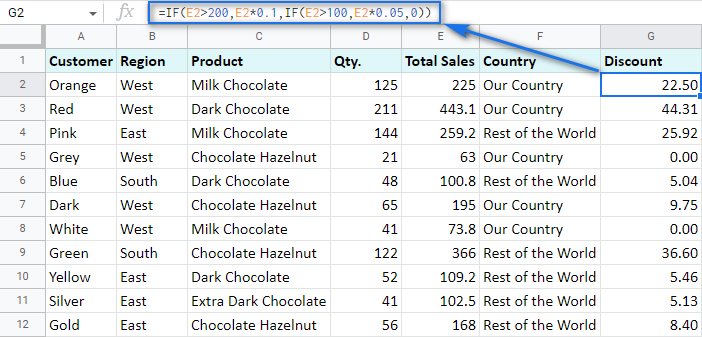Note that we used IF function as a second argument of the IF function. In this case, the decision tree is as follows:But what if we make it even more fun and complicate the task? Imagine that we're offering the discounted price to the one region only - "East".

To do that correctly, we add the logical expression AND to our function. The formula then will look the following way:

`=IF(AND(B2="East",E2>200),E2*0.1,IF(AND(B2="East",E2>100),E2*0.05,0))`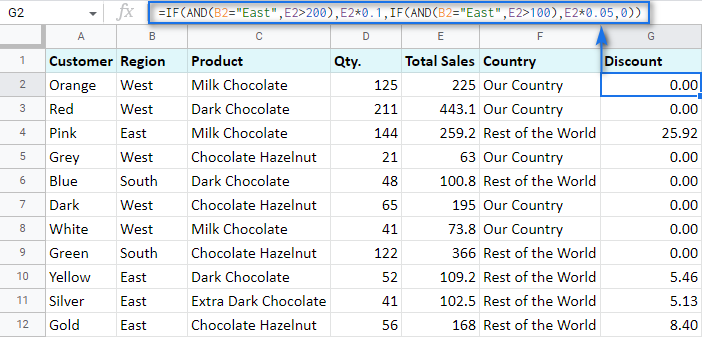As you can see, the number of discounts have reduced greatly while their amount remains intact.

We hope that there's no room for any doubt now that the IF function, though a very simple one at first glance, opens the door to many options to data processing in Google Sheets.

### 291 Responses to "Google Sheets IF function - usage and formula examples"

1.Patrick Price says:

MILES RATE TOTAL
34 1.88 \$366.22

How do i put a mileage range of numbers like this
miles rate
0 to 5.9 = 1.01
6 to 10.9 = 1.11
11 to 15.9 = 1.25
and so on in to a formula any help would be great.

•Natalia Sharashova (Ablebits.com Team) says:

Hello Patrick,

If I understand your task correctly, here's how you should put that down to the formula (supposing everything starts from A1):
=IF(AND(A2>=0,A2<=5.9),1.01,IF(AND(A2>=6,A2<=10.9),1.11,IF(AND(A2>=11,A2<=15.9),1.25,IF(AND(A2...),1.88,IF...))))

•Ben says:

Were you able to get this to work? I tried something similar and am returning an error message.

=IF(AND(D30>749,D301625,D30=2500),\$1500)))

•Natalia Sharashova (Ablebits.com Team) says:

Hi Ben,

You miss the comparison operator in your second condition: D301625
Also, I don't see the point of using AND to check whether D30 equals 2500 and is more than 749 at the same time. :) It is either equal to 2500 or not.

2.Lindsay says:

I'm trying to populate a certain text in column F if column c says a specific thing. I'm using =IF(C3:C237 = "DMM901Y", "No PPW Status Available", " ") on the F column, but it's not working. All help is appreciated. Thank you!

•Lindsay says:

Edit:: It works on a singular cell, but not the whole column. I want the rule to work for the whole column.

•Natalia Sharashova (Ablebits.com Team) says:

Thank you for your question, Lindsay.

Try wrapping your IF with an ARRAUFORMULA:
=ARRAYFORMULA(IF(C3:C237="DMM901Y","No PPW Status Available",""))

If you're wondering how it works, please check this blog post.

3.Mark says:

=IF(B2>=96.01%,"5",if(B2<96%,"4",if(B2<95%,3,IF(B2<94%,2,IF(B2<92%,1)))))
1 96.01%

4.s says:

If I want to use this function on existing data, and if the predicate is false I do not want the row to change, how do I go about that?

•Natalia Sharashova (Ablebits.com Team) says:

If I'm getting it correctly, just live the argument for the "false" result empty.

5.Aditya says:

How do we use the if statement to redirect to a website?
if value=true
then the person is redirected to a website.

•Natalia Sharashova (Ablebits.com Team) says:

Using the formula, you can only make it return a webpage for the user. Just enter the hyperlink as an argument, and it will appear if value=true. However, the person will have to click the link in order to open it.

6.michael says:

I would like that if A1 is 0 then put cell B1 in cell C1.
Is this possible?

•Natalia Sharashova (Ablebits.com Team) says:

Hello Michael,

yes, it is possible. Enter the following formula to your C1:
=IF(A1=0,B1,"")

•Lea says:

Is it possible to do this but searching for a value in a column?
I would like that if A1 = value in any cell in a column B, put cell C in the same row in cell D in same row.
Hope it makes scense.

7.Jackson says:

I am trying to add an IF statement that would add a multiplier if they play for a certain team. So like, I have rows of stats, name and team. So IF they play for the rockies they would have a .9375 multiplier. However the thing I need it multiplied to is already a formula. So can I add an if statement to a formula, if so, how?

•Natalia Sharashova (Ablebits.com Team) says:

Hello Jackson,

For me to be able to help you out, please share a small sample spreadsheet with us (support@4-bits.com) with your source data and the result you expect to get. I kindly ask you to shorten the table to 10-20 rows.
Note. That email for file sharing only. Please do not email there. Once you share the file, just confirm by replying here.

8.Christie says:

I am looking for help to create a formula that will calculate tax liability as follows:
1.) If annual income is less than \$42,900 then tax is calculated by multiplying the tax amount by 5.8%
2.) If annual income is \$42,900 or greater and is less than \$101,550 then tax is calculated by multiplying the difference between \$42,900 and the annual income by 6.5% then adding \$2,488.
3.) If annual income is greater than \$101,500 then tax is calculated by multiplying the difference between \$101,550 and the annual income by 7.15% and adding \$6,447

•Natalia Sharashova (Ablebits.com Team) says:

Hello Christie,

Please check if the formula below works as desired. It is written supposing that your tax amount is in column A and annual income is in column B, so just make the necessary adjustments based on your data:
=IF(B2<42900,A2*5.8%,IF(AND(B2>=42900,B2<101550),(B2-42900)*6.5%+2488,IF(B2>101500,(B2-101550)*7.15%+6447)))

9.Lady says:

Hello,
I'm trying to create a fixed daily summary of the data below.
Date Fruit Name Quantity
4-Nov-19 Apple 10
4-Nov-19 Orange 20
4-Nov-19 Grapes 50
4-Nov-19 Banana 15
5-Nov-19 Apple 20
5-Nov-19 Orange 50
6-Nov-19 Orange 20
6-Nov-19 Grapes 50
6-Nov-19 Banana 15
This below is the summary format I want to do
Summary
Date Apple Orange Grapes Banana
4-Nov-19
5-Nov-19
6-Nov-19
...

•Natalia Sharashova (Ablebits.com Team) says:

Hello,

I'm afraid IF is not the function you'd normally use to find matches.

You can either try the add-on described here to lookup matches by all conditions (date and fruit), or create a pivot table out of your source data.

10.Jerel says:

I'm doing of spread sheet of our household bills and want to show the difference from the previous month with some result being + change some being - change. How do i do that?

11.Nadeem says:

If(or(A1="",A1="TBD"),"",text(A1,"yyyy")))
Why this formula returns TBD if A1 contains TBD?

•Natalia Sharashova (Ablebits.com Team) says:

I'm sorry but the formula you provided can't return TBD in case it's already in A1. A cell with this formula will remain empty in this case.

12.Robert says:

I have a column that will a yes or no. Based on the answer, I would like for another column to calculate a tax. Example, if cell F13 is Yes, then I would like to look at an amount in cell G13 and calculate the tax based on the amount in cell G13. Then if cell F13 is No, I would like for it to look in cell G13 and calculate the tax based on the amount in cell G13. Bottom line, if a person is married (Yes) then there will be one calculation and if a person is not married (No) there will be a different calculation.

•Natalia Sharashova (Ablebits.com Team) says:

Hello Robert,

If I'm getting your task clear, a formula like this will do:
=IF(F13="Yes",calculation_for_YES_with_G13,calculation_for_NO_with_G13)

In case you're still not sure how it works, please describe how your data is arranged in more detail.

13.Jamie says:

Hello, I am trying to create an inventory tracker to track multiple items being sold at multiple locations. I have an Inventory sheet that has Product A listed (lets call that A2) and then a numerical quantity under Original Stock at Location A (B2). Then there's Current Stock at Location A (C2). Then I have a Sales sheet. What I'd like is when a sale is recorded for Product A at Location A, for C2 on the Inventory sheet to equal (B2- the new sale). I was able to make an IF formula that works when the sale is recorded in a specific cell, but the sales will be listed in chronological order so I'm trying to make the formula work for the entire column all the way down. Is there any way to do that? Thanks so much!

•Natalia Sharashova (Ablebits.com Team) says:

Hello Jamie,

I'm really sorry but the description is a bit confusing. Please share a small sample spreadsheet (support@4-bits.com) with your source data and the result you expect to get. I kindly ask you to shorten the tables to 10-20 rows.

Note. The email address above is for file sharing only, please do not email there. Once you share the file, just confirm by replying here.

I'll look into it and do my best do advise you.

14.cAYTI says:

Hi there, I am trying to create a ticket tracking system that will display pricing based on location and date. I have 5 locations named "1J", "3A", "4Y", "10Y", and "ND" that are listed using Data Validation in column C, and Dates in November are Listed in Column A. I would like to have the price populate in column B based on the Location selected AND if the date listed in column A is before 11/18/19 the price would show one amount, and if the same location was selected but it was after 11/18/19 then it show a different amount. Example: If "1J" was selected on 11/5/19 the price would be "\$20", If "1J" was selected on 11/19/19 the price would be "\$22" because 11/19/19 is after 11/18/18. I want cell B2 to change if the date is before 11/18/19 and a certain location has been chosen in cell C2. So far this formula works but how would I create a formula that allows for the other 4 locations to possibly be selected and location relevant pricing populate?
Also FYI 11/18/19=43787
=IF(AND(A2<43787,C2= "1J"), "\$18", "\$20")

•Natalia Sharashova (Ablebits.com Team) says:

Hello,

If I understand your task correctly and the prices vary not only based on dates but also on locations, you need to create a nested IF and list each price for each set of conditions, for example:
=IF(AND(C2="1J",A2<43787),"\$18",IF(AND(C2="1J",A2>=43787),"\$20",IF(AND(C2="3A",A2<43787),...))))

15.Madison says:

Hello, I am trying to make a sheet that connects to a form. The form will be asking what tools are needed, if they put needed i want it to put a 1 in the column and if its not needed than put a 0. How would i formulate that? i tried =if(B4 "needed", "1", "0") but that did not work.

TIA!

•Natalia Sharashova (Ablebits.com Team) says:

You missed an equal sign after the cell reference. Also, numbers don't need to be in quotes. Please try this formula:
=IF(B4="needed",1,0)

16.Eana Kathleen Gonzales says:

Hi, I'm trying to create a cell that will show if the date in one cell is "outdated" or "updated" and a blank return if there is no date listed on that specific cell. I have already create the first part, but I'm having a hard time in introducing the "BLANK" part. I'm new in this kind of stuff and still exploring. Hope you could help.
Thank you!

•Natalia Sharashova (Ablebits.com Team) says:

Hi Eana,

Use a pair of double quotes ("") to check if a cell is blank (=IF(A2="",...)) or to return a blank cell if the condition is met (=IF(A2=20,"YES",""))

17.Munna says:

Hi,
i wanted to make due date format based on three criteria. I wanted a texts on a specific cell like "Processing" if date on other cell is later than 7 days from today, "Coming soon" if date is within next 7 days from today and "Pending" is date is earlier than today. i used below formula but only for "coming son " criteria not working properly. cell shows "coming soon" if the date is one day earlier than today. others criteria working properly.

Formula used:
=IFS(I2<TODAY(),"Pending",I2TODAY()+7,"Processing")

•Natalia Sharashova (Ablebits.com Team) says:

Hi Munna,

You need to use AND for the "Coming soon" criteria:
AND(I2>=TODAY(),I2<TODAY()+7),"Coming Soon"

I'd recommend adding it for "Pending" as well to avoid getting it if I2 is empty:
AND(I2<TODAY(),I2<>""),"Pending")

18.JB says:

how can I input the value from the cell in a formula? for instance
=If(\$B2="Campbell", substitute(\$H2," [ ", " Campbell "),\$H2)
The value of B2 is Campbell but the value of C2 is another name. So rather than have to type the name manually or pasting it into the formula, how can I input the value from any cell into the formula above?

•Natalia Sharashova (Ablebits.com Team) says:

Hello JB,

I'm sorry, can you please provide examples of what is exactly in C2 (since you're looking for a square bracket) and what you need to have as a result of the formula?

19.Scott Foster says:

I pickup cars in different zones and each zone has a different pay rate. Is there an if formula to calculate each zone and pay rate on one sheet?

•Natalia Sharashova (Ablebits.com Team) says:

Hello Scott,

You can either build a nested IF and specify each zone and its pay rate there (they can be listed in an additional table and simply referenced in the formula) or use IFS instead.

20.Mike Darger says:

Principal:Interest:Balance:
\$330.29 \$69.71 \$23,465.76
\$83.32 \$131.68 \$23,382.44
\$587.18 \$27.82 \$22,795.26
\$606.29 \$8.71 \$22,188.97
\$536.55 \$78.45 \$21,652.42
\$0.00 \$21,652.42
I'm wanting the spreadsheet to show "\$0.00" if there is no principal or interest is added in the row. How would I do the formula to make it show this? =SUM(K10-I11) is what the formula is looking like!

•Natalia Sharashova (Ablebits.com Team) says:

Hello Mike,

I'm sorry I'm a bit confused. Is it the balance that should show \$0.00 if principal and interest are missing or something else? What do you use the SUM formula for?

Thank you.

21.DHOLE S. M. says:

i want to permit serially typing to 50 staff members in excel(google spread sheer) eg. 1.ABC, 2.DEF, 3.GHI, 4.JKL, in this serial other than 1 will not type data before 1st NO., then only No. 2 will type data, then only 3, then only 4 ---will type the data by using e-mail

•Natalia Sharashova (Ablebits.com Team) says:

Hello there,

I'm really sorry but your task is not clear to me. Please describe it in more detail and provide an example of what you have at the start and what you're trying to get.
I'll try my best to help.

22.SAYRA says:

If(N2,N2,if(O2,\$P\$1,""))
IN THIS FORMULA,EVERYTHING IS PERFECT. BUT THE PROBLEM IS THAT WHEN I ENTERED A GOOGLE FORM SHEET AND IT APPEAR RESULT ON GOOGLE SHEET. THE FORMULA AUTOMATICALLY SHIFTED INTO NEXT CELL.
PLEASE HELP ME: JUST TELL ME HOW I CAN ALLOCATE FORMULA EACH AND EVERY CELL IF ANOTHER INFO. IS ENTERED FROM GOOGLE FORM LATER...

•Natalia Sharashova (Ablebits.com Team) says:

Hello Sayra,

I'm afraid you will have to copy the formula manually since each submitted Form response is added as a new row to Google Sheets.

23.Tawana Deter says:

I'm trying to do a function where if F2, G2, or H2 are yes then I2:M2 are 0 but if F2, G2, or H2 are no then it's the autosum of I2:M2. I know this can be done in excel but the computer I have I am not able to put excel on. Can anyone please help me write this out to where it works or tell me if it can even be done?

•Natalia Sharashova (Ablebits.com Team) says:

Hello Tawana,

I guess this is a formula you need:
=IF(OR(F2="yes",G2="yes",H2="yes"),0,SUM(I2:M2))

However, you can't enter it to I2:M2 since these cells should be counted if any of F2, G2, or H2 have "yes" in them.
So in other words, either the formula should be entered to other cells or you need to adjust the conditions.

24.Jessie says:

=IF(and(B2="Wellness Pod","70")IF(and(B2="45min Pod Roll","47.40")))

B2 column is a drop menu that we select which service the client had.

I then need the value or a percentage of that value to display in the total column.

(Also this is in google sheets)

•Natalia Sharashova (Ablebits.com Team) says:

Hello Jessie,

If I'm getting it clear, your formula should look like this:
=IF(B2="Wellness Pod",70,IF(B2="45min Pod Roll",47.4,IF(...)))

25.Ricky says:

Hi,
I'm trying to write a formula that will give me a text statement in column B if column A contains a specific number.
For instance Column A can have a number that is 1-24, each number has a different item assigned to it. What I would like to do is put in the specific number in column A and have column B auto populate with the item name that correlates to it.
1 = Apple
2 = Orange
7 = Pumpkin
So on and so forth.
Is there a formula that will actually do this?

•Natalia Sharashova (Ablebits.com Team) says:

Hi Ricky,

Sure. Assuming your numbers start from A2 and end in A10, here's the formula for numbers you specified:
=ARRAYFORMULA(IF(A2:A10=1,"Apple",IF(A2:A10=2,"Orange",IF(A2:A10=7,"Pumpkin"))))

26.Dan says:

Hi,
I am trying to write a formula and was hoping someone may be able to help.
I have created a training matrix for my work.
Column A is the name of each employee, and Column B is labeled (Safety Training) Column C is labeled (ESD Training). Under each column I place an X next if the person in column A has been trained.

On a second sheet, I am making a page for (Safety Training). I want that page to list each name that has an X under the safety training column on Sheet 1.

I have created this formula =IF(Matrix!T6="X", Matrix!A6) which will bring the names into sheet 2, but In a list of 10 names, I see the names in column A on sheet 2, but the word "FALSE" elsewhere.

Is there a way to look at sheet 1, and take just the names in Column A, that also have an X in Column T and list them on Sheet 2?
I hope all of this makes sense.
Thanks for the help.

•Natalia Sharashova (Ablebits.com Team) says:

Hi Dan,

Your formula looks correct, so it's hard to tell why it doesn't work without seeing your data.

I should also mention that it is VLOOKUP that is usually used to match and pull records from one table to another. We also feature a special tool that returns all matches based on your conditions. Feel free to check it out.

27.Katrina says:

Hello!
I am working with a donation spreadsheet that lists the companies and the years 2017, 2018, 2019 in separate columns. In each column for the years, the amount each company donated is in those columns. Some companies donated one year and not the others while others donated all three years. Is there any way for me to sort or filter which companies donated in 2019, in all three years, and the companies who donated in 2017 & 2018 but not in 2019? Would a filter be the best option, or are there ways to do a table or chart of some sort to organize this data by who donated which years?

•Natalia Sharashova (Ablebits.com Team) says:

Hello Katrina,

The easiest way, to my opinion, is to try our special tool that works like an advanced filter: it will create different lists based on different conditions (empty/not empty) without interfering with your source data.

There are a few other standard ways to try though:

1. Filter columns by conditions to meet your criteria. However, you'll need to change filtering conditions a few times to see the donations over different years.
2. There are also options of creating a pivot table or a chart but you'll have to figure out how to set them properly for your task.
28.Sandeep says:

Hello
I am trying to create a formula but not able to crack it. There are 3 parameters based on which the result is calculated and also there is All parameter which is not there in the data. When I select All it should calculate for all the data and when i select a specific parameter is should calculate for that. I also need the result based on any combination. This is the formula i have come up with but it does not populate result If I select All for all the parameters.It does only for one selection of All
=IF(BD41="All",(SUMPRODUCT((\$AY\$2:\$AY\$6600=\$BD\$2)*(\$AO\$2:\$AO\$6600=BE41)*(\$B\$2:\$B\$6600=BF41)*(\$AQ\$2:\$AQ\$6600=BF42)*1)),IF(BE41="All",(SUMPRODUCT((\$AY\$2:\$AY\$6600=\$BD\$2)*(\$C\$2:\$C\$6600=BD41)*(\$B\$2:\$B\$6600=BF41)*(\$AQ\$2:\$AQ\$6600=BF42)*1)),IF(BF41="All",(SUMPRODUCT((\$AY\$2:\$AY\$6600=\$BD\$2)*(\$C\$2:\$C\$6600=BD41)*(\$AO\$2:\$AO\$6600=BE41)*(\$AQ\$2:\$AQ\$6600=BF42)*1)),(SUMPRODUCT((\$AY\$2:\$AY\$6600=\$BD\$2)*(\$AO\$2:\$AO\$6600=\$BE\$41)*(\$C\$2:\$C\$6600=BD41)*(\$B\$2:\$B\$6600=BF41)*(\$AQ\$2:\$AQ\$6600=BF42)* 1)))))

•Natalia Sharashova (Ablebits.com Team) says:

Hello Sandeep,

I'm really sorry but I'm afraid we won't be able to trap the error without seeing your data.

29.Tom says:

I am trying to create a If formula the if x=1 then pull results from another tab and cell. x could equal 1-5. When I creat this function: =if(F\$12=1,'Tier Monthly Incomes'!B2,if(F\$12=2,'Tier Monthly Incomes'!C2),if(F\$12=3,'Tier Monthly Incomes'!D2),if(F\$12=4,'Tier Monthly Incomes'!E2),if(F\$12=5,'Tier Monthly Incomes'!F2)) .

I am getting an error message that says, "Wrong number of arguments to IF. Expected between 2 and 3 arguments, but got 6 arguments." Can you think of a way that I could make this work or suggest an alternative way to address this? Thanks!

•Natalia Sharashova (Ablebits.com Team) says:

Hi Tom,

The problem is that you put a comma and each new IF after the closing bracket, while they should go inside of those, like the very first one you built. In other words, you need to move three first closing brackets to the very end of your formula:
=IF(F\$12=1,'Tier Monthly Incomes'!B2,IF(F\$12=2,'Tier Monthly Incomes'!C2,IF(F\$12=3,'Tier Monthly Incomes'!D2,IF(F\$12=4,'Tier Monthly Incomes'!E2,IF(F\$12=5,'Tier Monthly Incomes'!F2)))))

30.JASON BURKETT says:

I am creating a Workbook for my organization and am trying to pull data from different sheets into another sheet. I understand how to do that but I want one cell to pull data on the page from a different sheet, but then a different cell on that same page to read the data from the first cell and then pull the data from another sheet. I hope that makes sense.

•Natalia Sharashova (Ablebits.com Team) says:

Hello Jason,

I described different ways of pulling data between sheets in this blog post. You can also incorporate them to your IF function to check some condition first and then pull the needed data based on it.

31.Cindi Nance says:

Is there a way to make this to not spit out false if the cell is blank? We would like to keep adding to this spreadsheet without a running list of FALSE down column K, if that makes sense.
=if(F3="Chace: xxx","@ChaceChilds",if(F3="James: xxx","@JamestheRich"))

•Natalia Sharashova (Ablebits.com Team) says:

Hello Cindi,

Sure. It is double quotes that are used to indicate a blank cell. If I'm getting your task right, try adding the pair as the third parameter at the very end of your IF:
=IF(F3="Chace: xxx","@ChaceChilds",IF(F3="James: xxx","@JamestheRich",""))

32.Victor Hristov says:

Hello, I am trying to sum several cells into another cell. Just by reading this, you would say "oh, that's easy". Not as simple as it is though. My aim is to have 3 options in a cell and each option should equal to a specific number (eg. D2 cell has options "Red" which is 0, "Yellow" which is 1, and "Green" which is 2. So the text in the cell should equal a number. I have several of these cells to create and all of them should be summed into another cell (eg. D2,E2,F2 to be all summed into J2). Hope it's understandable. :-)

•Natalia Sharashova (Ablebits.com Team) says:

Hello Victor,

Just to get it clear: are all 3 words in one cell? Or are they in different cells that you need to sum eventually? Also, are there any other words in cells?
Please provide the exact contents of all the cells that you'd like to sum.
Thank you.

33.Chris says:

I am trying to calculate break times out of hours worked, but my break times change depending on how many hours you work in a day - e.g work more than 6h10m but less than 8h10m = 30 min, any higher and it's 45 min.
My formula is
=IF(OR(E3>TIME(6,10,0),E3TIME(8,10,0),E3<TIME(9,9,0)),E3-TIME(0,45,0)))
I have tried adding a the second "IF" argument, but it keeps returning only the 30 min deduction even when the result is greater than the 8h10min and I am not sure where it is going wrong. Am I asking too much of the formula for the one cell?

•Natalia Sharashova (Ablebits.com Team) says:

Hello Chris,

Will this formula do what you need?
=IF(AND(E3>=TIME(6,10,0),E3<=TIME(8,10,0)),E3-TIME(0,30,0),IF(E3>TIME(8,10,0),E3-TIME(0,45,0),""))

The first part checks if the time spent falls between 6h10m and 8h10m. If so, it subtracts 30 min from E3. The second IF checks if more than 8h10m was spent, in which case 45 min are subtracted.
The very last double quotes mean that a cell will remain empty if it took less than 6h10m for the job.

34.David says:

Hi! I'm trying to make a formula that will return a numerical value (1 or .25) based on the information in 2 cells. If a cell has "trainer" in it, then return ".25", and if a cell has "sponsored", then return "1".
I tried using the if(and formulas listed above, but get an error message. Here's my formula for reference.
I also tried a different approach with nested if statements, and can get a result of 1 for sponsored, but get a blank cell for trainer. Here's that formula for reference.
Any ideas?

•Natalia Sharashova (Ablebits.com Team) says:

Hi, David,

You missed a comma right before 0.25 (after the closing bracket) in your first formula, that's why it fires an error.
As for the second one, the closing bracket after 0.25 is an excess one.

35.kevin says:

Hey I am trying to populate my table using the if statement where I would like to check the value of a Boolean expression from column I so that if the statement returns as correct the value of column H should appear as the same in Column J So how should I write the statement

•Natalia Sharashova (Ablebits.com Team) says:

Hello Kevin,

I believe a formula like this should help:
=ARRAYFORMULA(IF(I2:I10=TRUE,J2:J10,""))

36.Peg Allegretto says:

If number in cell E2 equals 1-3, cell G2 equals \$0.
If number in cell E2 equals 4-6, cell G2 equals \$100.00
If number in cell E2 equals 7-9, cell G2 equals \$250.00
If number in cell E2 equals 10 or more, cell G2 equals \$500.00

Can you help me with this please?
Thank you! Peg

•Natalia Sharashova (Ablebits.com Team) says:

Hello Peg,

You need to create a nested IF and introduce the AND operator for your conditions, like this:
=IF(AND(E2>=1,E2<=3),0,IF(AND(E2>=4,E2<=6),100,IF(AND(E2>=7,E2<=9),250,IF(E2>=10,500,""))))

37.Lea says:

Is it possible to do this but searching for a value in a column?
I would like that if A1 = value in any cell in a column B, put cell C in the same row in cell D in same row.
Hope it makes scense.

38.Sebastian Keil says:

Hi,
how would I put If field A bigger than field B then x, otherwise y.
=IF(C3>A2,"Achtung","ok")
doesn't work.
Thanks

•Natalia Sharashova (Ablebits.com Team) says:

Hi Sebastian,

try checking your spreadsheet locale, perhaps you should use other delimiters than commas. It is explained in details here.

39.Jonny says:

Hi, great article, but I'm still struggling a little with this and am hoping you could help, please! I've created a dropdown with 5 options (Option A, Option B, Option C, etc.). If I select Option A in my dropdown, I want to display multiple cell values (x15) using QUERY. I can get this to work if I pick one option only using this:

Picking 'Option A' in the dropdown will return the correct data. However, I can't work out how to chain together an IF statement so if Option B is selected, then I QUERY a different part of Spreadsheet 1, or if I select Option C, it queries a yet another part of the spreadsheet etc.

Any suggestions on how to link these together would be super helpful! Or even being told that it's not possible would be useful!

40.Kris says:

I'm trying to get a function that if a score is greater than 31 it says "exceeds" and turns blue, 22-28 it says "meets" and turns green, 14-21 it says "approaches" and turns yellow orange, and <=13 it says "Does Not Meet" and turns red but having some issues getting it to do all of that at once.

•Natalia Sharashova (Ablebits.com Team) says:

Hello Kris,

you're right, you can't do it all at once.

To make cells return a certain text, you need to build a nested IF in those cells. You will find how to do that in the last paragraph of the blog post above.

As for colours, you will have to create conditional formatting rules with the same IF formulas for each colour.

•Kris says:

Okay, I'm still having trouble with the nested IF statements though. Specifically the ones that are between two values like 14-21.

41.Rachel says:

I have a tracking sheet for projects with dollar amounts. Is there a way to do it that if the checkbox is checked (which in Google, it's marked TRUE) in cell A1, then the \$ amount listed in cell F1 is added to the Total amount in cell F10. But if the checkmark isn't checked (FALSE), then that's not added to the Total in F10.
All my brain can handle is: =IF(A1"TRUE" ... it's mush after that.
Thanks!